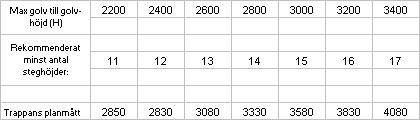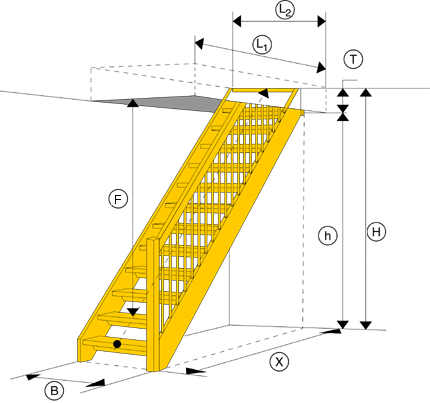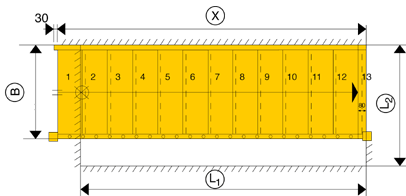# Required space and important measures for a straight staircase.

How much space does a straight staircase require?

Description:
The step depth is 250 mm, measured in the tread line, which is located in the middle of the stair tread width.
The measurements shown below include the end tread of 80 mm but not the 30 mm nosing of the string at the start.

How to calculate:

1. Measure your total height from floor below to floor upstairs (H)
2. Choose the number of rises. (For example, if you have a floor height of 2650 mm, you choose 14 step heights.)
3. Choose the width of the staircase. (B).
4. The measurement you will find in the table is the horizontal length (X) of the stair.
For example, on a straight staircase with 14 step heights, the outer dimension (X)=3330.How to measure a straight staircase?
Designations

H = Stair height – measured vertically from the floor below to the floor upstairs
h = Room height – measured from the floor below to the
T = Well thickness.

(With the measurements of room height and vault thickness, you can calculate the clearance at the beginning of the stairs.)

B = The total staircase width. .
X = The horizontal measurement of the first flight of the staircase.
Y = The horizontal measurement of the second flight of the staircase.
Z = The horizontal measurement of the third flight of the staircase.

L1 = The horizontal measurement of the well opening at the X-measurement of the staircase.
L2 = The horizontal measurement of the well opening at the Y-measurement of the staircase.

(It doesn’t matter if you have plenty of space for the stairs downstairs.
The size of the stairwell – and the height of the room – determines how large the stairs you can have.)